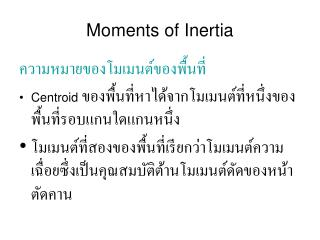DownloadDownload PresentationMoments of Inertia

# Moments of Inertia

Télécharger la présentation## Moments of Inertia

- - - - - - - - - - - - - - - - - - - - - - - - - - - E N D - - - - - - - - - - - - - - - - - - - - - - - - - - -
##### Presentation Transcript

1. Moments of Inertia ความหมายของโมเมนต์ของพื้นที่ • Centroid ของพื้นที่หาได้จากโมเมนต์ที่หนึ่งของพื้นที่รอบแกนใดแกนหนึ่ง • โมเมนต์ที่สองของพื้นที่เรียกว่าโมเมนต์ความเฉื่อยซึ่งเป็นคุณสมบัติต้านโมเมนต์ดัดของหน้าตัดคาน

2. โมเมนต์ที่สองของพื้นที่โมเมนต์ที่สองของพื้นที่ • Stress within the beam varies linearly with the distance from an axis passing through the centroid C of the beam’s cross-sectional area σ = kz • For magnitude of the force acting on the area element dA dF = σ dA = kz dA

3. Definition of Moments of Inertia for Areas • Since this force is located a distance z from the y axis, the moment of dF about the y axis dM = dF = kz2 dA • Resulting moment of the entire stress distribution = applied moment M • Integral represent the moment of inertia of area about the y axis

4. Moment of Inertia • Consider area A lying in the x-y plane • Be definition, moments of inertia of the differential plane area dA about the x and y axes • For entire area, moments of inertia are given by

5. Moment of Inertia • Formulate the second moment of dA about the pole O or z axis • This is known as the polar axis where r is perpendicular from the pole (z axis) to the element dA • Polar moment of inertia for entire area,

6. Parallel Axis Theorem for an Area • For moment of inertia of an area known about an axis passing through its centroid, determine the moment of inertia of area about a corresponding parallel axis using the parallel axis theorem • Consider moment of inertia of the shaded area • A differential element dA is located at an arbitrary distance y’ from the centroidal x’ axis

7. The fixed distance between the parallel x and x’ axes is defined as dy • For moment of inertia of dA about x axis • For entire area • First integral represent the moment of inertia of the area about the centroidal axis

8. Second integral = 0 since x’ passes through the area’s centroid C • Third integral represents the total area A • Similarly • For polar moment of inertia about an axis perpendicular to the x-y plane and passing through pole O (z axis)

9. Moments of Inertia for an Area by Integration Example 10.1 Determine the moment of inertia for the rectangular area with respect to (a) the centroidal x’ axis, (b) the axis xb passing through the base of the rectangular, and (c) the pole or z’ axis perpendicular to the x’-y’ plane and passing through the centroid C.

10. Solution Part (a) • Differential element chosen, distance y’ from x’ axis • Since dA = b dy’

11. Solution Part (b) • Moment of inertia about an axis passing through the base of the rectangle obtained by applying parallel axis theorem

12. Solution Part (c) • For polar moment of inertia about point C

13. โมเมนต์อินเนอร์เชียของพื้นที่ประกอบโมเมนต์อินเนอร์เชียของพื้นที่ประกอบ Example 10.5 Compute the moment of inertia of the composite area about the x axis.

14. Solution Composite Parts • Composite area obtained by subtracting the circle form the rectangle • Centroid of each area is located in the figure

15. Solution Parallel Axis Theorem • Circle • Rectangle

16. Solution Summation • For moment of inertia for the composite area,

17. Example 10.6 Determine the moments of inertia of the beam’s cross-sectional area about the x and y centroidal axes.

18. Solution Composite Parts • Considered as 3 composite areas A, B, and D • Centroid of each area is located in the figure

19. Solution Parallel Axis Theorem • Rectangle A

20. Solution Parallel Axis Theorem • Rectangle B

21. Solution Parallel Axis Theorem • Rectangle D

22. Solution Summation • For moment of inertia for the entire cross-sectional area,